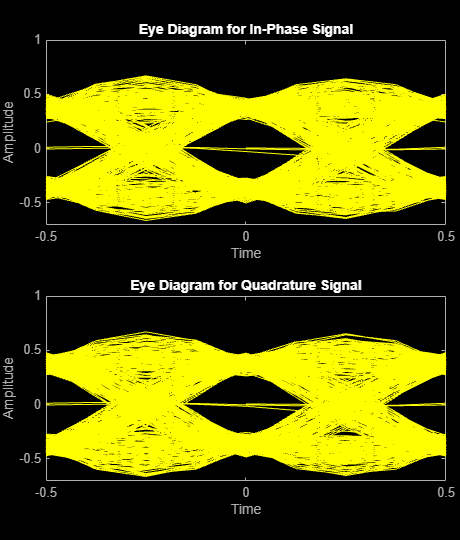# eyediagram

Generate eye diagram

## Syntax

``eyediagram(x,n)``
``eyediagram(x,n,period)``
``eyediagram(x,n,period,offset)``
``eyediagram(x,n,period,offset,plotstring)``
``eyediagram(x,n,period,offset,plotstring,h)``
``h = eyediagram(___)``

## Description

````eyediagram(x,n)` generates an eye diagram for signal `x`, plotting `n` samples in each trace. The labels on the horizontal axis of the diagram range between –1/2 and 1/2. The function assumes that the first value of the signal and every `n`th value thereafter, occur at integer times.```
````eyediagram(x,n,period)` sets the labels on the horizontal axis to the range between –`period`/2 to `period`/2.```
````eyediagram(x,n,period,offset)` specifies the offset for the eye diagram. The function assumes that the (`offset` + 1)th value of the signal and every `n`th value thereafter, occur at times that are integer multiples of `period`.```
````eyediagram(x,n,period,offset,plotstring)` specifies plot attributes for the eye diagram.```

example

````eyediagram(x,n,period,offset,plotstring,h)` generates the eye diagram in an existing figure whose handle is `h`. NoteUse of `hold on` to plot multiple signals in the same figure is not supported. ```
````h = eyediagram(___)` returns the handle to the figure that contains the eye diagram. You can specify any of the input argument combinations from the previous syntaxes.```

## Examples

collapse all

Generate an eyediagram of a filtered QPSK signal.

Generate random symbols. Apply QPSK modulation to get a modulated signal.

```data = randi([0 3],1000,1); modSig = pskmod(data,4,pi/4);```

Specify the number of output samples per symbol parameter. Create a transmit filter object, `txfilter`.

```sps=4; txfilter = comm.RaisedCosineTransmitFilter('OutputSamplesPerSymbol',sps);```

Filter the modulated signal `modSig`.

`txSig = txfilter(modSig);`

Display the eye diagram.

`eyediagram(txSig,2*sps)`## Input Arguments

collapse all

Input signal, specified as a vector or matrix.

The interpretation of `x` and the number of plots depend on the shape and complexity of `x`.

• If `x` is a real-valued two-column matrix, the function interprets the first column as in-phase components and the second column as quadrature components. The two components appear in different subplots of a single figure window.

• If `x` is a complex-valued vector, the function interprets the real part as in-phase components and the imaginary part as quadrature components. The two components appear in different subplots of a single figure window.

• If `x` is a real-valued vector, the function interprets the vector as a real signal. The figure window contains a single plot.

Data Types: `double`
Complex Number Support: Yes

Number of samples per trace, specified as a integer greater than 1.

Data Types: `double`

Trace period, specified as a positive scalar. The labels on the horizontal axis of the eye diagram range between –`period`/2 to `period`/2.

Data Types: `double`

Offset value, specified as an integer in the range 0 to (`n`–1). The function assumes that the (`offset` + 1)th value of the signal and every `n`th value thereafter, occur at times that are integer multiples of the input `period`.

Data Types: `double`

Plot attributes, specified as a character vector or string scalar containing symbols.

This argument sets the plotting symbol, line type, and color for the eye diagram. The format and meaning of the symbols are the same as in the `plot` function. For example, the default value `'b-'` produces a solid blue line.

Data Types: `char` | `string`

Figure handle to an existing figure that contains an eye diagram, specified as a `Figure` object. `h` must be a handle to a figure that the `eyediagram` function previously generated.

## Output Arguments

collapse all

Figure handle, returned as a `Figure` object. To modify properties of this object, see Figure Properties.

## Version History

Introduced before R2006a